Name: ___________________Date:___________________

 Email us to get an instant 20% discount on highly effective K-12 Math & English kwizNET Programs!

### MEAP Preparation - Grade 4 Mathematics1.41 Types of Fractions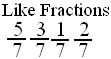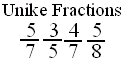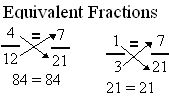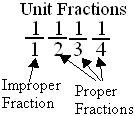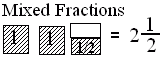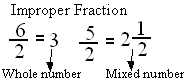Directions: Answer the following questions. Also write at least 5 examples of each type of fractions.
 Q 1: A fraction less than 1 is called a proper fraction. A fraction equal to one and more than 1 is called _________.proper fractionmixed fractionimproper fraction Q 2: When a products obtained by cross multiplication give the same number, then the fractions are ____(or equal).propermixedequivalent Q 3: An improper fraction is sometimes a mixed number or sometimes a whole number.FalseTrue Q 4: A mixed number denotes the sum of a whole number-part and proper fraction part.FalseTrue Q 5: If the numerator is less than the denominator in a fraction, the fraction is called a _______.improper fractionmixed fractionproper fraction Q 6: If the numerator is more than the denominator in a fraction, the fraction is called a _________.improper fractionmixed fractionproper fraction Q 7: Fraction with numerator 1 is called a unit fraction. Are all unit fractions proper?NoYes Q 8: Fractions with same denominators are calledunlike fractionslike fractions Question 9: This question is available to subscribers only! Question 10: This question is available to subscribers only!